# Catch up on the latest AI articles# Did You Beat BiGAN In Image Generation? About Diffusion Models

3 main points
✔️ Diffusion Models beat SOTA's BiGAN in generating highly accurate images
✔️ Explore the good architecture of Diffusion Models through a large number of ablation experiments and techniques
✔️ Control the balance between fidelity and diversity of the generated data with Diffusion Models

written by Prafulla DhariwalAlex Nichol
(Submitted on 11 May 2021 (v1), last revised 1 Jun 2021 (this version, v4))

Subjects: Machine Learning (cs.LG); Artificial Intelligence (cs.AI); Computer Vision and Pattern Recognition (cs.CV); Machine Learning (stat.ML)code：The images used in this article are from the paper, the introductory slides, or were created based on them.

## first of all

Over the years, generative models have been able to produce human-level text (How To Get The Real Value Out Of GPT-3 : Prompt Programming), highly accurate images (Pre-trained GAN Model To Super-resolution Technology), or human-like speech and music. However, there is a need for further research beyond the current SOTA model, and a wide range of fields such as graphic design games It is expected to be used in a wide range of fields such as graphic design, games, and music production.

In the current evaluation metrics where generative data quality is emphasized, GANs have obtained the main SOTA．On the other hand, GANs are prone to model collapse during training, and the low diversity of the generated data is a problem.

In this article, we introduce a paper that claims that the Diffusion Model, which is a different approach from GAN, has finally surpassed GAN.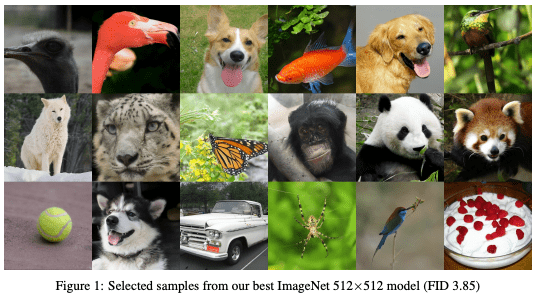Figure 1. shows an example of an image generated by the Diffusion Model, showing that it can generate indistinguishable images as well as GANs. the Diffusion Model generates data by gradually removing noise from a given signal. dataset, but still lacks accuracy on difficult datasets such as ImageNet.

The authors hypothesize that there are two differences between the Diffusion Model and the GAN: first, the Diffusion Model is not as architecturally explored as the GAN, and second, the GAN can control the trade-off between diversity and fidelity in the generated data. Taking these two points into account, we find a good architecture for the Diffusion Model through a large number of experiments and show that it can beat BiGAN, which is the current SOTA.

## Background of Diffusion Model

The Diffusion Model generates an image by gradually removing noise from a given signal. In fact, it starts from the noise x_T and goes to x_(T-1),x_(T-2),...,x_0. The Diffusion Model is trained to predict the noise. x_t is considered to be x_0 plus the noise t times.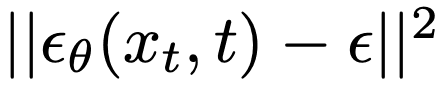Also, in this paper, all noise is assumed to be Gaussian noise, so the noise to be eliminated to move from each x_t to x_(t-1) can be expressed by the following equation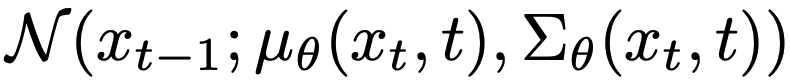where µ is the mean of the Gaussian noise and sigma is the variance, both are approximated by neural networks in this study. Please refer to the appendix for more details about the model.

## model

### Architecture Improvements

Based on the UNet architecture, which has been shown to be effective in previous studies, we conducted an architectural search focusing on the following areas. We focused on the following aspects of the architecture: the depth and breadth of the model, the number of heads and different resolutions of the attention mechanism, and the residual blocks used in BiGAN. Specifically, we will conduct controlled experiments to determine the values these should take.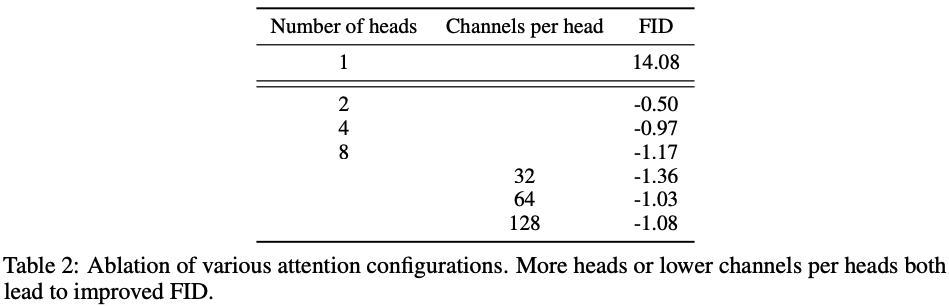From Table 2. more Heads is better and less channels gives better (lower) FID.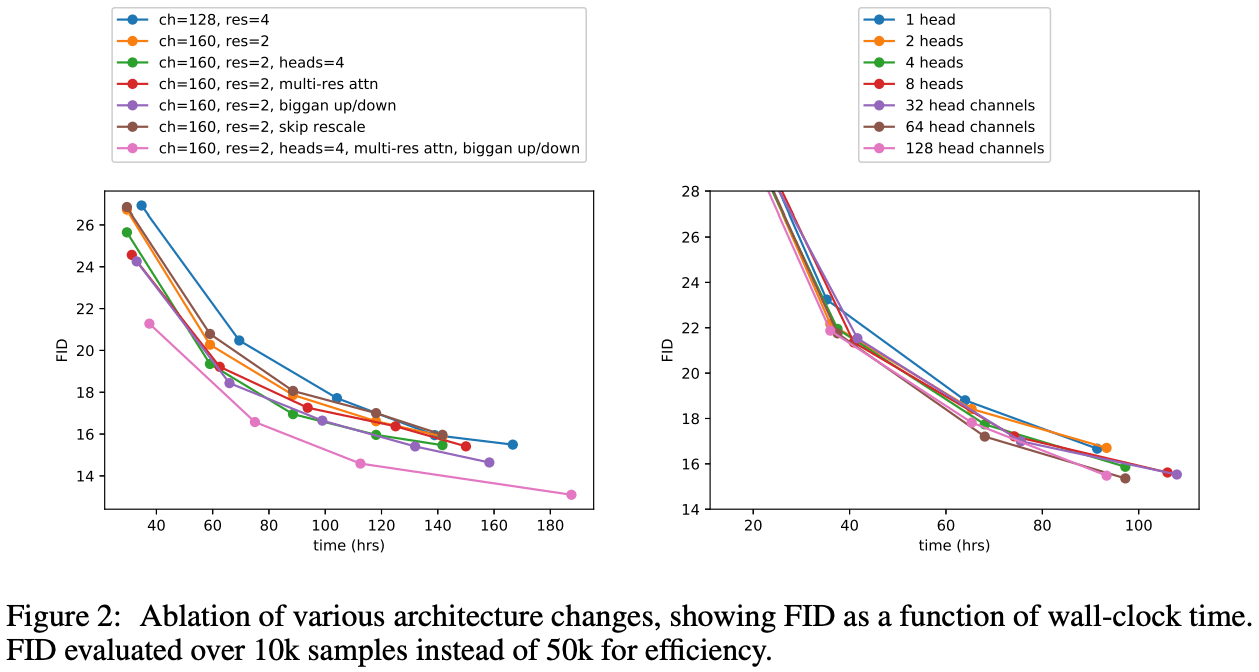The results in Figure 2 (left) show that increasing the number of residual blocks (res=4, blue) reduces the FID value, but it takes more training time to achieve the same level of accuracy. The pink line shows that the same level of accuracy can be obtained in a shorter training time and that a lower FID can be obtained in the end. As for the heads, we adopt 64 heads from the result of Figure 2. In subsequent experiments, we will use the best architecture found in this experiment for comparison.

### Classifier Guidance

In Algorithm 1 and 2, we present algorithms for conditioning Diffusion Models with stochastic process assumption and Diffusion Models with deterministic Diffusion Models with stochastic process assumption, and Diffusion Models with deterministic sampling assumption, respectively, by using a label classifier.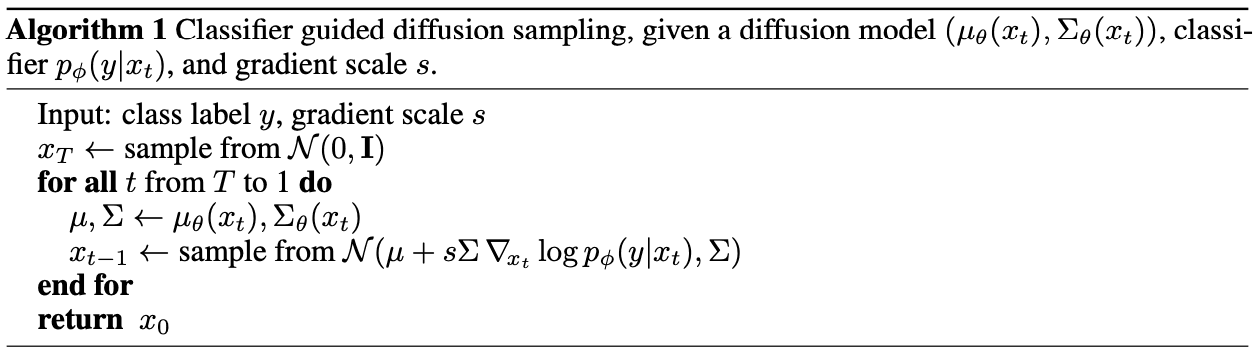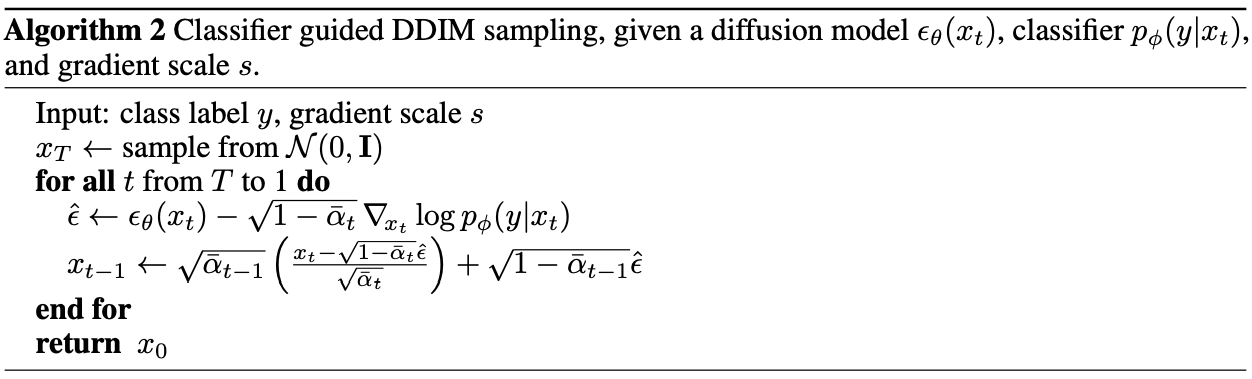A Gaussian distribution is assumed in Diffusion Models with general stochastic processes as in Algorithm 1. Label classifier conditioning is consistent with shifting the Gaussian distribution in proportion to the gradient of the classifier. On the other hand, in the deterministic sampling process, as shown in Algorithm 2, the Diffusion Models themselves incorporate the gradient of the classifier. For the detailed derivation, please refer to Section 4 of the paper. In fact, in this paper, we use Algorithm 1. and UNet trained on ImageNet as the classifier. In addition, the hyperparameter s can control the balance between accuracy and diversity of the generation.

So far, we have used classifier gradients to condition on Diffusion Models, but it is of course possible to directly train conditioned Diffusion Models. Furthermore, it is possible to utilize classifier gradients for conditioning Diffusion Models in a similar way.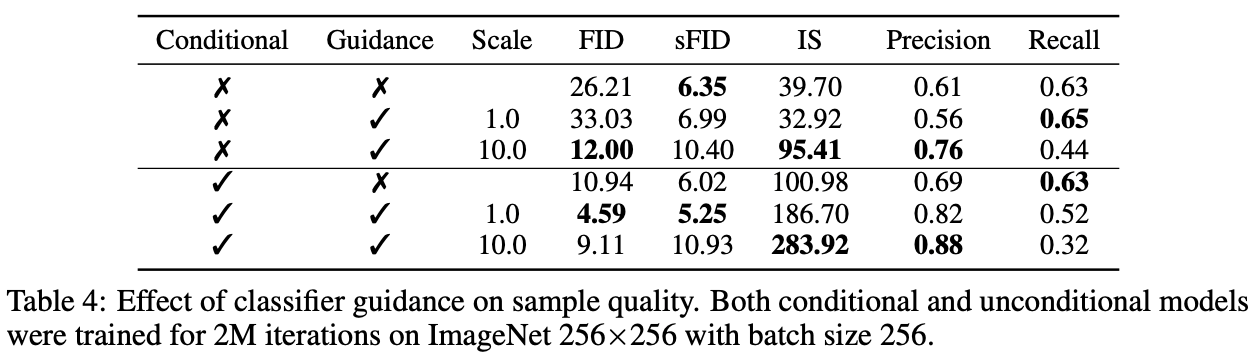## Table 4. summarizes the effects of Conditional Diffusion Models, which are trained with label conditions, and Classifier Diffusion Models, which use the gradient of the classifier. However, it sacrifices some diversity.

In Table 4, we summarize the effects of Conditional Diffusion Models, which are trained with label conditions, and Classifier Diffusion Models, which use the gradient of the classifier. The results show that the Conditional Diffusion Models have lower FID and better accuracy but at the expense of diversity.

Also, simply using the gradient of the classifier is equivalent to using the Conditional classifier only in terms of FID.

Furthermore, we can see that the accuracy is better when both are taken into account. We can also see that the hyperparameters adjust the balance between Precision and Recall.

## Comparison experiments

The validation experiments for the architecture optimized in this study were performed on the Bedrooms, Horses, and Cats classes of the LSUN dataset for the Ordinary Diffusion Model and on images of different resolutions in ImageNet for the Conditional Diffusion Model.

Table 5. summarizes the main results. The proposed method reaches the best FID in all experiments, and the sFID metric is the best except for ImageNet 64x64.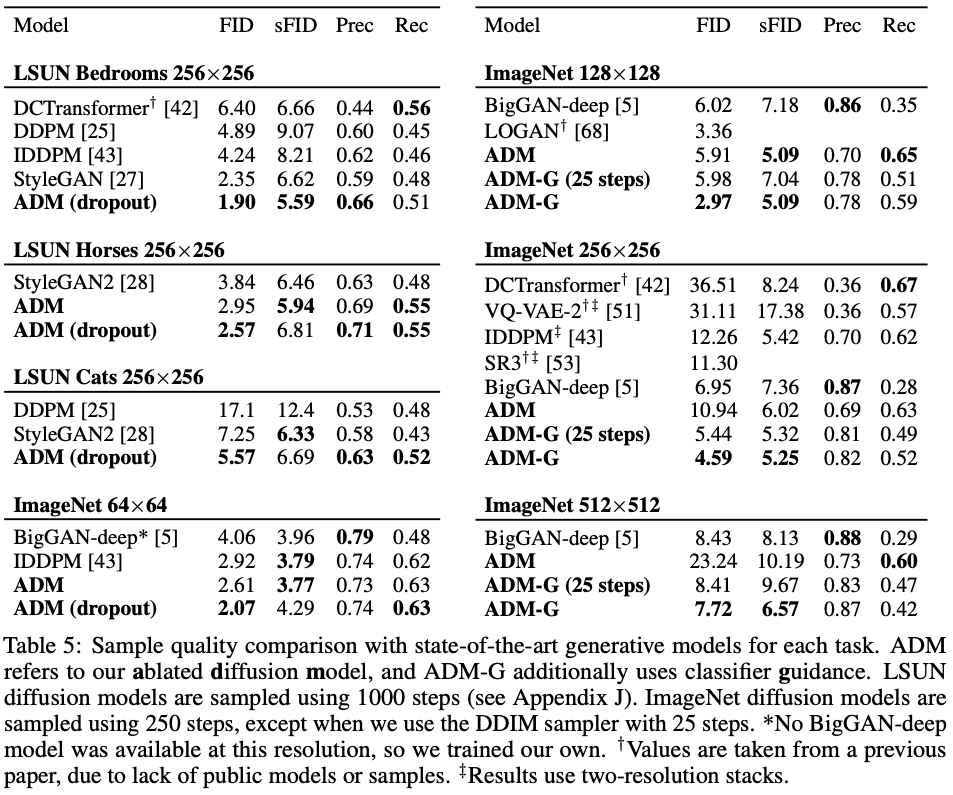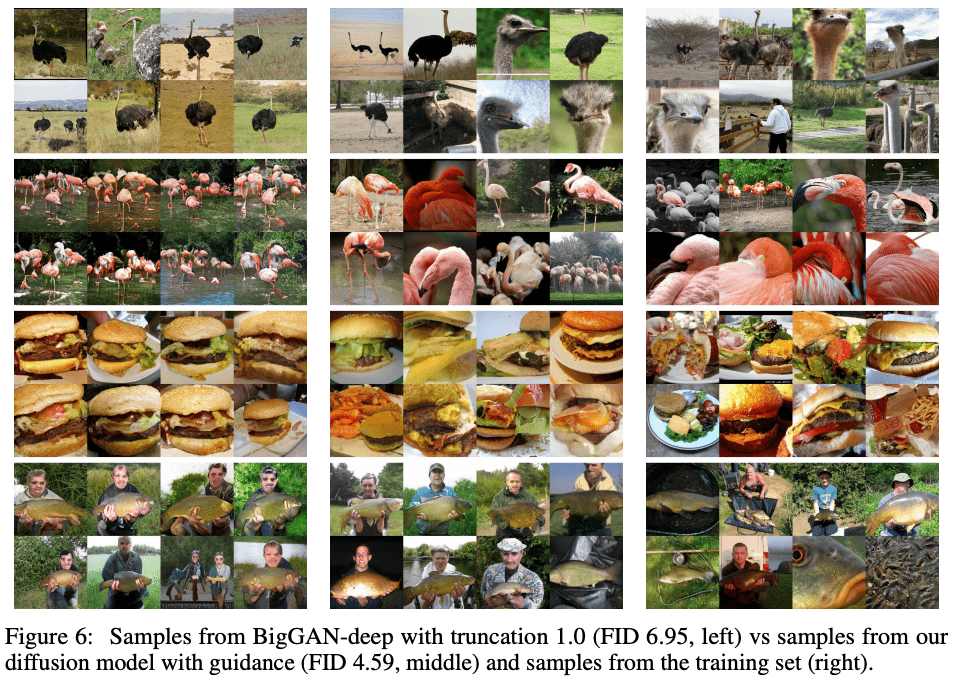Figure 6. shows the generated samples (left), the proposed method (center), and the training samples of BiGAN-deep with comparable FID. It can be seen from the samples that the proposed method can generate more diverse samples.

## summary

In this study, we have shown that Diffusion Models can generate samples with better accuracy (FID) than the SOTA model GANs. First, we searched for an architecture, following the work of GANs. Next, we used a technique that uses the gradient of the classifier in addition to the efficient architecture obtained. We found that by adjusting the gradient of the classifier, we can control the balance between fidelity and diversity of the generated images.

In this article, I introduced Diffusion Models, which are promising but have not received as much attention as GANs in the field of image generation, where GANs are leading. I also hope that subsequent research on Diffusion Models will be as exciting as that on GANs, which is why I introduced them here.

If you have any suggestions for improvement of the content of the article,